# Research on ride comfort of vibratory rollers: Part-1 Study on the effect of different cab isolation mounts

## Yan Kang1, Vanliem Nguyen2, Xiaoyan Guo3, Yuehan Li4

1, 2, 3, 4School of Mechanical and Electrical Engineering, Hubei Polytechnic University, Huangshi, 435003, China

1, 2, 3, 4Hubei Key Laboratory of Intelligent Conveying Technology and Device, Hubei Polytechnic University, Huangshi, 435003, China

2Corresponding author

Journal of Mechatronics and Artificial Intelligence in Engineering, Vol. 2, Issue 1, 2021, p. 1-10. https://doi.org/10.21595/jmai.2021.21860
Received 6 January 2021; received in revised form 21 January 2021; accepted 29 January 2021; published 5 February 2021

Copyright © 2021 Yan Kang, et al. This is an open access article distributed under the Creative Commons Attribution License, which permits unrestricted use, distribution, and reproduction in any medium, provided the original work is properly cited.
Views 80
Abstract.

In order to evaluate the ride quality of vibratory roller’s cab used the different isolation mounts including the traditional rubbers, hydraulics, and hydro-pneumatics, a nonlinear dynamics model of the vibratory roller under interaction of the deformable terrain and drum has been established based on Matlab/Simulink software. The root-mean-square (RMS) of the accelerations of the vertical driver’s seat and the cab’s pitch angle have been used as objective studies. The influence of damping and stiffness coefficients of different isolation mounts on the cab’s ride quality has been respectively simulated and analyzed under a hard ground at an excitation 28 Hz of the vibration drum. The research results indicate that the characteristics of non-linear damper and high-static stiffness of the hydro-pneumatic mounts can greatly reduce the vertical driver’s seat vibration and cab shaking when compared to vibratory roller’s cab using the traditional rubber mounts and hydraulic mounts under stiffness and damping coefficients of the vehicle and cab isolations. Particularly with the dynamics parameters of ${K}_{c}=$ [0.8 to 1.2]×${K}_{c0}$, ${C}_{c}=$ [0.8 to 1.0]×${C}_{c0}$, [0.6 to 1.0]×${K}_{t0,d0}$, and ${C}_{t,d}=$ [1.0 to 1.2]×${C}_{t0,d0}$.

Highlights
• Three different isolation mounts including the traditional rubbers, hydraulics, and hydro-pneumatics are researched to improve the vibratory roller ride comfort.
• The influence of damping and stiffness coefficients of three different isolation mounts on the cab’s ride quality has been respectively simulated and analyzed under a hard ground at an excitation 28 Hz of the vibration drum.
• The research results indicate that the characteristics of non-linear damper and high-static stiffness of the hydro-pneumatic mounts can greatly reduce the vertical driver's seat vibration and cab shaking when compared to vibratory roller's cab using the traditional rubber mounts and hydraulic mounts under stiffness and damping coefficients of the vehicle and cab isolations.

Keywords: vibratory rollers, ride comfort, rubber mount, hydraulic mount, hydro-pneumatic mount, off-road terrains.

#### 1. Introduction

The soil compactor often works in factories and construction site, and its operation process includes a combined static and dynamic forces of all the vehicle and the drum to compress the asphalt, terrain, or other materials. The vibration excitations from the deformable terrain and the drum are thus transmitted the driver via the cab and the vehicle's isolation systems. Consequently, the isolation system of the cab is one of the most important indexes for the improvement of the vehicle's ride quality.

Basic studies of the interaction between the wheel and deformable terrain [1-4] and of interaction between the elastic/plastic ground and drum  showed that the response in the vibration process of the vehicle had been strongly affected by terrains. Almost the isolation mounts of the cab of vibratory rollers were equipped by the traditional rubber with its high-stiffness coefficient and low-damping coefficient of the rubber materials [5, 6]. Thus, the high-stiffness of the rubber helped suppress only noise and vibration in the high frequency region, on the contrary, the low-damping coefficient of the rubber could generate the vibration with its high-magnitudes to reduce the ride quality of the vehicle . The effect of designed dynamic parameters of the rubber mounts of the cab on the ride quality had been evaluated . An optimal design for the cab’s new rubber mounts of soil compactors was built via the finite element model, and the new rubber mount was then produced and tested for the vehicle’s cab . The dynamic parameters of the rubber mounts of the cab had been also optimized for improving the ride quality . The research results concluded that the vehicle’s ride quality was significantly improved. But, the study results also showed that the large-amplitude shock of the cab existed at low-frequency region in the direction of forwarding motion. This is one of the reasons causes tiredness of the driver while working, cracking surfaces of the cab and other problems. Thus, to solve this problem, the low damping coefficients of the cab’s rubber mounts (RM) needs to be improved.

The hydraulic mounts (HM) were investigated and applied on the cab isolations of industrial vehicle, construction equipment, and earth-moving machinery to reduce the vibrations from the vehicle frame transmitted the cab floor and improve the ride quality [9, 10]. A cab model of off-road soil compactors equipped with the HM was also studied under the random excitation of the rigid road surface in a low-frequency range [11, 12]. The results showed that the ride comfort was greatly improved by hydraulic mounts. Additionally, the hydro-pneumatic mounts (HPM) researched for agricultural tractors and dump trucks to enhance the ride comfort as well as reduce the energy [13, 14] were applied on the cab’s isolation mounts of the vibratory rollers for improving the moving quality of the vehicle [15, 16]. Researches indicated that the ride quality of the cab had been strongly increased. However, the analysis the effect of the dynamic parameters of both the HM and HPM to understand their characteristics on the vibration isolation has not been researched yet.

In this study, a dynamics model based on the actual vibratory rollers is built under the vibration excitations consisted by the drum/tires-deformable terrain contact in the condition of the vehicle traveling; and of an excitation of the drum 28 Hz in the condition of the vehicle working in an elastic/plastic ground. The characteristics of the RM, HM, and HPM on the vibratory roller ride comfort are then analyzed compared via the root-mean-square (RMS) of the acceleration responses of the vertical driver’s seat and cab’s pitch angle, respectively. The improvement of the ride quality and control of the cab shaking is this study aim.

#### 2. The dynamics model for the vibratory roller

Vibratory roller has been mainly composed of our parts: vibratory steel drum, vibration excitation mechanism, vibration isolation system, and walking mechanism. The vibrator drum is not only a working wheel but also a walking wheel. It is one of the important parts of vibrating roller. Its weight accounts for about 80 % of the total vibration. The vibration damping system is composed of multiple shock absorbers which are used for vibration isolation and vibration reduction connection between vibration wheel and frame. The nonlinear dynamic model of vehicle is modeled as in Fig. 1, where, ${z}_{b}$, ${z}_{1}$, ${z}_{c}$, and ${z}_{s}$ are the vertical displacement of the rear frame, front frame, cab, and seat; ${z}_{d}$ is the vertical displacement of the flat section of the drum-ground contact surface; ${m}_{b}$, ${m}_{1}$, ${m}_{c}$, and ${m}_{s}$ are the weights of the rear frame, front frame, cab, and seat; ${I}_{b}$ and ${I}_{c}$ are the moment of inertia of the rear frame and the cab; ${k}_{s}$, ${k}_{c1}$, and ${k}_{c2}$ are the stiffness of the vibration isolators of the seat, front and rear cab, respectively; ${c}_{s}$, ${c}_{c1}$, and ${c}_{c2}$ are the damping of the vibration isolators of the seat, front and rear cab, respectively; ${q}_{t1}$ is excitation of the road roughness; ${\varphi }_{c}$ and ${\varphi }_{b}$ are the cab and rear frame's pitch angles; ${l}_{1}$, ${l}_{2}$, ${l}_{3}$, ${l}_{4}$, ${l}_{5}$, ${l}_{6}$, and ${l}_{7}$ are the geometric dimensions.

Fig. 1. The nonlinear dynamics model of vibratory rollers with different cab isolations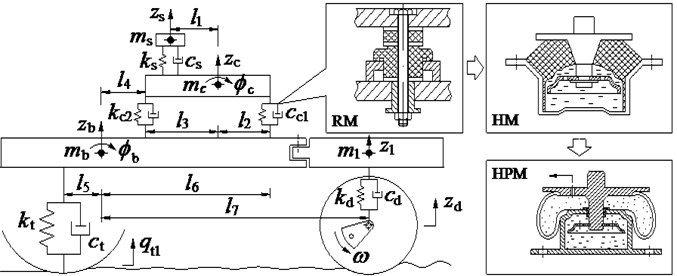Based on the model of the vibratory roller dynamics in Fig. 1, the different dynamic equations of the vehicle are listed as follows:

(1)
${m}_{s}{\stackrel{¨}{z}}_{s}={c}_{s}\left({\stackrel{˙}{z}}_{c}+{\stackrel{˙}{\varphi }}_{\mathrm{c}}{l}_{1}-{\stackrel{˙}{z}}_{s}\right)+{k}_{s}\left({z}_{c}+{\varphi }_{\mathrm{c}}{l}_{1}-{z}_{s}\right),$
(2)
${m}_{c}{\stackrel{¨}{z}}_{c}={c}_{c1}\left({\stackrel{˙}{z}}_{b}+{\stackrel{˙}{\varphi }}_{\mathrm{c}}{l}_{2}-{\stackrel{˙}{\varphi }}_{b}{l}_{6}-{\stackrel{˙}{z}}_{c}\right)+{k}_{c1}\left({z}_{b}+{\varphi }_{\mathrm{c}}{l}_{2}-{\varphi }_{b}{l}_{6}-{z}_{c}\right),$
(3)
${m}_{1}{\stackrel{¨}{z}}_{1}={c}_{d}\left({\stackrel{˙}{z}}_{d}-{\stackrel{˙}{z}}_{1}\right)+{k}_{d}\left({z}_{d}-{z}_{1}\right),$
(4)
${m}_{b}{\stackrel{¨}{z}}_{b}={c}_{t}\left({\stackrel{˙}{q}}_{t1}-{\stackrel{˙}{\varphi }}_{b}{l}_{5}-{\stackrel{˙}{z}}_{b}\right)+{k}_{t}\left({q}_{t1}-{\varphi }_{b}{l}_{5}-{z}_{b}\right)=0,$
(5)
${I}_{c}{\stackrel{¨}{\varphi }}_{\mathrm{c}}={l}_{3}\left[{c}_{c2}\left({\stackrel{˙}{z}}_{b}-{\stackrel{˙}{z}}_{c}-{\stackrel{˙}{\varphi }}_{\mathrm{c}}{l}_{3}-{\stackrel{˙}{\varphi }}_{b}{l}_{4}\right)+{k}_{c2}\left({z}_{b}-{z}_{c}-{\varphi }_{\mathrm{c}}{l}_{3}-{\varphi }_{b}{l}_{4}\right)\right]$

(6)
${I}_{b}{\stackrel{¨}{\varphi }}_{b}={l}_{5}\left[{c}_{t}\left({\stackrel{˙}{q}}_{t1}-{\stackrel{˙}{z}}_{b}-{\stackrel{˙}{\varphi }}_{b}{l}_{5}\right)+{k}_{t}\left({q}_{t1}-{z}_{b}-{\varphi }_{b}{l}_{5}\right)\right]$

#### 3. Modeling of Cab isolation systems

To evaluate the effect of the lumped parameters of the different isolation mounts of the cab including the RM, HM, and HPM, as plotted in Fig. 1, their mathematical models are then given in Fig. 2.

Fig. 2. The model of the different cab isolations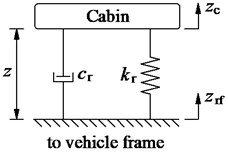a) Rubber mount (RM)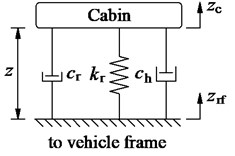b) Hydraulic mount (HM)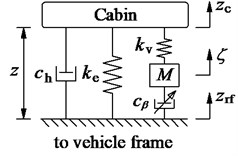c) Hydro-pneumatic mount (HPM)

With the RM, as plotted in Fig. 1 and Fig. 2(a), it is made of rubber and metal and it can effectively isolate vibration by absorbing vibration energy. It is defined by a low damping coefficient ${c}_{r}$ and high stiffness ${k}_{r}$. The corresponding vertical dynamic forces of the front and rear RMs are given as follows:

(7)

With the HM, as plotted in Fig. 1 and Fig. 2(b), it includes a main rubber material and a damper with its damping coefficient ${c}_{h}$ used to isolator the vibration of the rear vehicle frame. Its mathematical equation is defined as follows [8, 9]:

(8)

With the HPM, as shown in both Fig. 1 and Fig. 2(c), it includes an airbag used together with a damper to isolator the vibrations. In the mathematical model of Fig. 2(c), herein, ${k}_{v}$ and ${k}_{e}$ are the coefficients of the HPM’s linear stiffness, ${c}_{\beta }$ is the main damping parameter of the pneumatic mount, and ${c}_{h}$ is the variable damping coefficient.

The corresponding vertical dynamic forces of the front and rear HPM are given as follows :

(9)
(10)
$\left\{\begin{array}{l}{z}_{ci}={z}_{ci}+\left(-1{\right)}^{i+1}{\varphi }_{c}{l}_{i+1},\\ {\stackrel{˙}{z}}_{ci}={\stackrel{˙}{z}}_{ci}+\left(-1{\right)}^{i+1}{\stackrel{˙}{\varphi }}_{c}{l}_{i+1}.\end{array}\right\$
(11)

where, ${z}_{ci}$ and ${z}_{rfi}$ in Eqs. (7-9) are the vertical displacements of suspension of cab and front and rear frames, $i=$ 1, 2.

#### 4.1. The interaction model between the elastic/plastic soil and drum

Based on the result of Adam and Kop [2, 3], the interaction model between the elastic/plastic soil and drum has been built in Fig. 3, and the properties of the elastic and plastic of the soil ground have been expressed by the factors $\epsilon$ and $\gamma$ as follows:

(12)

where, the parameters of $\epsilon$, ${k}_{se}$, ${k}_{sp}$, and ${c}_{se}$ are the compression ratio, elastic stiffness, compression stiffness, and compression damper.

Fig. 3. The drum and elastic-plastic interaction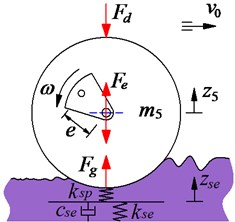Based on the research results of Nguyen et al. [8-9], the interaction between the drum and deformable soil ground has been defined by three phases including loading phase, unloading phase, and drum-hope phase. The dynamic equations of three phases based on Refs. [7, 8] has been rewritten as follows:

(13)
(14)
${m}_{5}{\stackrel{¨}{z}}_{5}={F}_{dj}-{c}_{se}{\stackrel{˙}{z}}_{5}+{m}_{e}e{\omega }^{2}\mathrm{s}\mathrm{i}\mathrm{n}\omega t,$
(15)

Eqs. (13-15) have been then used to calculate the motion of the drum ${z}_{5}$ and the excitation forces ${F}_{d}$.

#### 4.2. The wheels-road surface roughness

Based on the ISO proposal , the terrain surface roughness $q\left(t\right)$ has given by $S\left(\mathrm{\Omega }\right)=S\left({\mathrm{\Omega }}_{0}\right)\left(\mathrm{\Omega }/{\mathrm{\Omega }}_{0}{\right)}^{-{w}_{0}}$. Where ${w}_{0}=$ 3 with $\mathrm{\Omega }\le {\mathrm{\Omega }}_{0}$, ${w}_{0}=$ 2.25 with $\mathrm{\Omega }>{\mathrm{\Omega }}_{0}$, $S\left({\mathrm{\Omega }}_{0}\right)$ is the random terrain determined via the reference frequency of ${\mathrm{\Omega }}_{0}=$ 1/2$\pi$ (cycle.m-1).

Additionally, when the vehicle moving with a speed ${v}_{0}$ on the rough road, its road surface is then calculated via the series:

(16)
$q\left(t\right)={\sum }_{i=1}^{N}{s}_{i}\mathrm{sin}\left(i\mathrm{\Delta }\omega t+{\phi }_{i}\right),$

where $N$ is the number of intervals, ${s}_{i}=\sqrt{2S\left(i\mathrm{\Delta }n\right)\mathrm{\Delta }n}$ is the amplitude excitation harmonic, $S$ is the target spectral density, $∆n=2\pi /L$.

Based on the results of Mitschke , the excitation of the off-road terrain can be yielded by selecting a value in the spectral density ranges.

#### 5. Simulations and discussions

At present, the evaluation of vehicle vibration is mainly divided into two categories including subjective and objective evaluation. Due to the complexity of people’s subjective feelings, psychological and physiological characteristics need to be taken into account, and specialized personnel are needed to carry out the evaluation, so the subjective evaluation is subject to certain limitations. The objective evaluation method mainly considers the vibration isolation performance of the vehicle. The physical quantity of mechanical vibration (frequency, amplitude, acceleration, etc.) is taken as the evaluation index. Therefore, this objective evaluation is a widely used method. Vibration acceleration is the basic parameter for the ride comfort evaluation. According to the weighted root-mean-square (RMS) method of vibration acceleration recommended by ISO-2631, the RMS acceleration is calculated according to the following formula:

(17)
${a}_{wz}=\sqrt{\frac{1}{T}{\int }_{0}^{T}{a}_{z}^{2}\left(t\right)dt},$

where, $T$ is the sampling time and $a\left(t\right)$ is the acceleration in the simulation porcess.

When vibratory rollers is moving or compressing on off-road grounds, the parameters of the stiffness and damping of the cab isolations, wheels, drum’s isolations not only affect the stability, durability and safety, but also impact the vehicle’s ride quality. Thus, the model of the vibratory roller is simulated to analyze the acceleration responses of the vertical driver's seat and the cab pitch angle with three cab isolations of the RM, HM, and HPM under different operation conditions of the vibratory roller.

#### 5.1. Influence of the parameters of the cab isolations

To evaluate the performance of the cab isolations of the vibratory roller, based on the lumped parameters of the vibratory roller and cab isolation mounts in Tables 1 and 2, a single-drum vibratory roller working at the excitation frequency of the drum 28 Hz and 5 km/h on a hard soil ground is simulated. The simulation results of the acceleration responses of the vertical driver’s seat and pitching cab angle are plotted in Fig. 4.

The results show that the acceleration responses of the vertical driver and pitching cab angle with the HPM is the smallest while that of the RM is the biggest. Therefore, the vehicle’s ride comfort is strongly affected by the cab isolation system. To analyze the influence of the dynamic parameters of the isolations of the on the ride quality, the influence of the stiffness and damping parameters of the RM, HM, and HPM on the vibration of the vertical driver’s seat and the cab shaking will being researched.

Table 1. The parameters of the vibratory rollers

 Parameter Value Parameter Value Parameter Value Parameter Value ${m}_{s}$ / kg 85 ${I}_{cy}$ / kgm2 523 ${l}_{4}$ / m 0.136 ${k}_{d}$/ N m-1 3.9×106 ${m}_{c}$ / kg 891 ${I}_{by}$ / kgm2 1.2×104 ${l}_{5}$ / m 0.76 ${k}_{t}$ / N m-1 0.5×106 ${m}_{b}$ / kg 2822 ${l}_{1}$ / m 0.383 ${l}_{6}$ / m 0.9 ${c}_{s}$ / Ns m-1 1.2×102 ${m}_{1}$ / kg 4464 ${l}_{2}$ / m 0.1 ${l}_{7}$ / m 0.6 ${c}_{d}$ / Ns m-1 2.9×103 ${m}_{d}$ / kg 4378 ${l}_{3}$ / m 0.524 ${k}_{s}$ / N m-1 1.2×104 ${c}_{t}$ / Ns m-1 4.0×103

Table 2. The parameters of the cab isolation mounts

 Parameter Value Parameter Value Parameter Value ${k}_{r1}$ / Nm-1 9.1×105 ${k}_{v1}$/Nm-1 15.3×105 ${c}_{\beta 1}$ / Ns2m-2 12.4×103 ${k}_{r2}$ / Nm-1 1.2×105 ${k}_{v2}$/Nm-1 2.01×105 ${c}_{\beta 2}$ / Ns2m-2 10.7×103 ${M}_{1}$ / kg 98 ${c}_{r1}/$Nsm-1 218 ${c}_{h1}$ / Ns2m-2 20×103 ${k}_{e1}$ / Nm-1 9.1×105 ${c}_{r2}$/Nsm-1 29 ${c}_{h2}$ / Ns2m-2 4.5×103 ${k}_{e2}$ / Nm-1 1.2×105 ${M}_{2}$/kg 33 $\epsilon$ 0.87

Table 3. The parameters of the elastoplastic ground with its hard soil

 Parameter Value Parameter Value ${k}_{sp}$ / N m-1 283×106 ${\omega }_{0}$ 2.14 ${c}_{se}$ / Ns m-1 37.1×103 $S\left({m}_{0}\right)$ / m3 cyc-1 3782.5×10-6

Fig. 4. The acceleration responses under a hard soil ground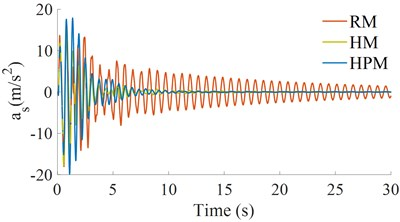a) The vertical driver’s seat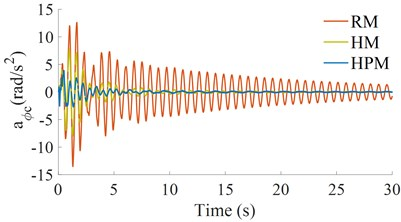b) The pitching cab angle

#### 5.1.1. Influence of the stiffness parameters

Under the same simulation condition of $v=$ 5 km/h of the vehicle and $f=$ 28 Hz of the drum on the hard soil ground, the different stiffness of the cab isolations ${K}_{c}=$ [0.2, 0.4, …, 2.0]×${K}_{c0}$with the RM, HM, and HPM is selected to simulate the result. The effect of the different stiffness of the suspension system of the vehicle on the RMS acceleration responses of the vertical driver's seat (${a}_{wz}$) and pitching cab angle (${a}_{w\varphi c}$) is shown in Fig. 5.

Fig. 5. The RMS acceleration responses under the different stiffness coefficients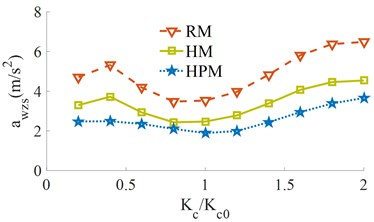a) The vertical driver’s seat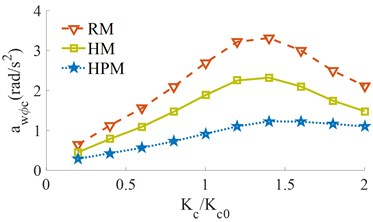b) The pitching cab angle

The RMS acceleration response of the vertical driver’s seat (${a}_{wz}$) in Fig. 5(a) shows that when the stiffness of the cab isolations is increased from 0.2×${K}_{c0}$ to 0.8×${K}_{c0}$, the ${a}_{wz}$ is slightly reduced. When the stiffness value is increased from 1.2×${K}_{c0}$ to 2.0×${K}_{c0}$, the ${a}_{wz}$ is strongly increased. With the stiffness values from 0.8×${K}_{c0}$ to 1.2×${K}_{c0}$, the ${a}_{wz}$ with all three RM, HM, and HPM is the smallest. This means that the vertical vibration of the driver’s seat is greatly improved. Besides, the RMS acceleration response of the pitching cab angle (${a}_{w\varphi c}$) in Fig. 5(b) shows that when the stiffness of the cab isolations is increased from 0.2×${K}_{c0}$ to 1.4×${K}_{c0}$, the ${a}_{w\varphi c}$ is strongly increased. When the stiffness value is increased from 1.4×${K}_{c0}$ to 2.0×${K}_{c0}$, the ${a}_{w\varphi c}$ is significantly reduced. With the stiffness value of ${K}_{c}=$ 1.4×${K}_{c0}$, the ${a}_{w\varphi c}$ is the bigest. Thus, the cab shaking is also the biggest. The comparison results between the RM, HM, and HPM in Fig. 5 show that both the ${a}_{wz}$ and ${a}_{w\varphi c}$ with the HPM is the smallest under the different stiffness coefficients. Therefore, the vehicle’s ride comfort is greatly improved by using the HPM. Additionally, a stiffness range of ${K}_{c}=$ [0.8 to 1.2]×${K}_{c0}$ should be chosen to further improve the vehicle’s ride comfort and control the cab shaking.

#### 5.1.2. Influence of the damping parameters

The different damping’s of ${C}_{c0}=$ [0.2, 0.4, …, 2]×${C}_{c0}$ with three various isolations of the RM, HM, and HPM are simulated under the same simulation condition of Section 5.1.1. The simulation results are shown in Fig. 6.

The result shows that both the ${a}_{wz}$ and ${a}_{w\varphi c}$ with the RM is insignificanlty changed with the different damping coefficients of the RM. This is due to the ${c}_{r}$ value of the RM is very small. With the change of the ${c}_{h}$ values, both the ${a}_{wz}$ and ${a}_{w\varphi c}$ are significantly reduced when increasing the ${c}_{h}$ values, thus, the driver’s ride comfort and cab shaking is also improved by the increasing damping coefficient of the HM.

With the HPM, when the damping value is increased from 0.5×${C}_{c0}$ to 1.0×${C}_{c0}$, both the ${a}_{wz}$ and ${a}_{w\varphi c}$ are slightly reduced and smaller than that of the HM. Conversely, with the damping value of the HPM increased from 1.0×${C}_{c0}$ to 2.0×${C}_{c0}$, both the ${a}_{wz}$ and ${a}_{w\varphi c}$ are strongly increased and highter than that of the HM, thus, the vehicle’s ride comfort is greatly reduced. Based on the analysis results, the damping range of ${C}_{c}=$ [0.8 to 1.0]×${C}_{c0}$ should be chosen to further improve the vehicle’s ride comfort and control the cab shaking with the HPM.

Fig. 6. The RMS acceleration responses under the different damping coefficients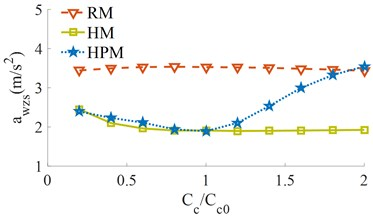a) The vertical driver’s seat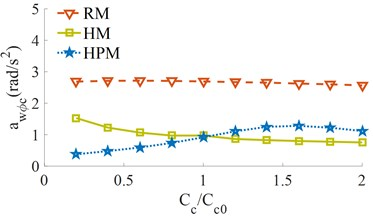b) The pitching cab angle

#### 5.2.1. Influence of the stiffness parameters

Under the same simulation condition of $v=$ 5 km/h of the vehicle and $f=$ 28 Hz of the drum on the hard soil ground, the stiffness effect of the tires and drum isolations ${K}_{t,d}=$ [0.2, 0.4, …, 2.0]×${K}_{t0,d0}$ on the ${a}_{wz}$ and ${a}_{w\varphi c}$is also obtained, as shown in Fig. 7.

Fig. 7. The RMS acceleration responses under the different stiffness coefficients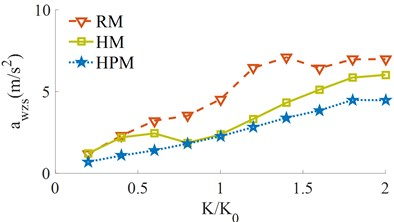a) The vertical driver’s seat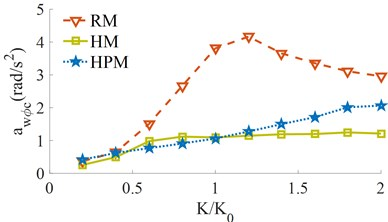b) The pitching cab angle

The simulation results indicate that both the ${a}_{wz}$ and ${a}_{w\varphi c}$ with all the RM, HM, and HPM are quickly increased with the increase of the stiffness coefficient of both the tires and drum isolations from 0.2×${K}_{t0,d0}$ to 2.0×${K}_{t0,d0}$. Thus, the vertical driver’s ride comfort and cab shaking is greatly reduced. To improver the vehicle’s ride comfort, the stiffness value of the tires and drum isolations should be chosen by ${K}_{t,d}=$ [0.6 to 1.0]×${K}_{t0,d0}$. Additionally, the performance of the HPM on the improvement of the vehicle’s ride comfort is insignificantly affected under the different stiffness values of the tires and drum isolations.

#### 5.2.2. Influence of the damping parameters

Similarly, the effect of the different damping’s of the tires and drum isolations ${C}_{t,d}=$ [0.2, 0.4, …, 2.0]×${C}_{t0,d0}$ on the ${a}_{wz}$ and ${a}_{w\varphi c}$ is simulated under the same condition of $v=$ 5 km/h of the vehicle and $f=$ 28 Hz of the drum on the hard soil ground. The simulation results are shown in Fig. 8.

The simulation results show that both the ${a}_{wz}$ and ${a}_{w\varphi c}$ with all the RM, HM, and HPM are significantly affected by the dampings of the tires and drum isolations. When the damping coefficients of the tires and drum isolations are increased from 0.2×${C}_{t0,d0}$ to 2.0×${C}_{t0,d0}$, both the ${a}_{wz}$ and ${a}_{w\varphi c}$ with both the RM and HM are reduced. However, the comparison result between the HM and HPM shows that with ${C}_{t,d}=$ [0.2 to 1.0]×${C}_{t0,d0}$ and ${C}_{t,d}=$ [1.4 to 2.0]×${C}_{t0,d0}$, the ${a}_{wz}$ of the HPM is higher than the HM, thus, the vehicle's ride comfort is reduced by using the HPM. To improve the vehicle’s ride comfort and cab shaking, the damping coefficients of the tires and drum isolations should be also chosen by ${C}_{t,d}=$ [1.0 to 1.2]×${C}_{t0,d0}$.

Fig. 8. The RMS acceleration responses under the different damping coefficients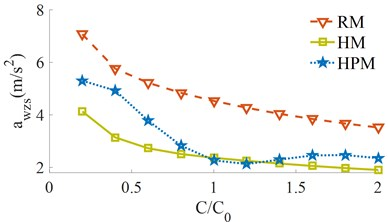a) The vertical driver’s seat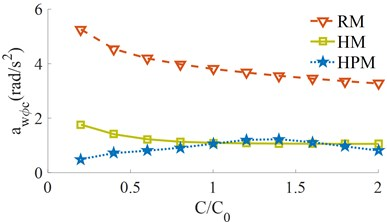b) The pitching cab angle

#### 6. Conclusions

Based on the simulation results of the effect of the vehicle and cab isolations’ parameters on the improvement of the ride comfort and cab shaking with three cab isolations of the RM, HM, and HPM in this paper, a conclusion can be drawn:

1) The different dynamic parameters of the vehicle and cab isolations have significant effect on the ride comfort of the vibratory roller.

2) Under the different simulation conditions of the dynamic parameters, the vehicle’s ride comfort and cab shaking with the HPM is better improved compared to both the RM and HM.

3) To optimize the vehicle’s ride comfort, the stiffness and damping parameters of the cab isolations, tires, and drum isolations should be designed in a range of ${K}_{c}=$ [0.8 to 1.2]×${K}_{c0}$, ${C}_{c}=$ [0.8 to 1.0]×${C}_{c0}$, ${K}_{t,d}=$ [0.6 to 1.0]×${K}_{t0,d0}$, and ${C}_{t,d}=$ [1.0 to 1.2]×${C}_{t0,d0}$.

4) To further improve the vehicle's ride comfort and control the cab shaking, the HPM with the damping coefficient of the hydraulic mount will be studied and controlled in Part-2.

#### Acknowledgements

This research was supported by Open Fund Project of Hubei Key Laboratory of Intelligent Transportation Technology and Device, Hubei Polytechnic University, China (No. 2020XY105) and Talent Introduction Fund Project of Hubei Polytechnic University (No. 19XJK17R), and Research Project of Hubei Polytechnic University, China (No. 18XJZ05Q).

1. Bekker M. Introduction to Terrain-Vehicle Systems. University of Michigan Press, Ann Arbor, USA, 1969. [Search CrossRef]
2. Wong J. Theory of Ground Vehicles. John Wiley and Sons, New York, NY, USA, 2001. [Search CrossRef]
3. Mitschke M. Dynamik Der Kraftfahrzeuge. Springer-Verlag, Berlin, Germany, 1972. [Publisher]
4. Nguyen V., Zhang J., et al. Effect of the off-road terrains on the ride comfort of construction vehicles. Journal of Southeast University, Vol. 35, Issue 2, 2019, p. 191-197. [Search CrossRef]
5. Nguyen V., Zhang J., et al. Vibration analysis and modeling of an off-road vibratory roller equipped with three different cab’s isolation mounts. Shock and Vibration, Vol. 2018, 2018, p. 8527574. [Publisher]
6. Li J. Q., Zhang Z. F., Xu H. G., Feng Z. X. Dynamic characteristics of the vibratory roller test-bed vibration isolation system: Simulation and experiment. Journal of Terramechanics, Vol. 56, 2014, p. 139-156. [Publisher]
7. Nguyen V., Zhang J., et al. Ride quality evaluation of the soil compactor cab supplemented the auxiliary hydraulic mounts via simulation and experiment. Journal of Central South University, Vol. 35, 2019, p. 273-280. [Search CrossRef]
8. Nguyen V., Nguyen K. Enhancing the ride comfort of the off-road vibratory roller cab by adding damper hydraulic mount. Vibroengineering Procedia, Vol. 21, 2018, p. 89-95. [Publisher]
9. Jiao S. J., Wang Y., Zhang L., Hua H. Shock wave characteristics of a hydraulic damper for shock test machine. Mechanical Systems and Signal Processing, Vol. 24, 2010, p. 1570-1578. [Publisher]
10. Lee P., Vogt J., Han S. Application of hydraulic body mounts to reduce the freeway hop shake of pickup truck. SAE Technical Paper Series, 2009-01-2126, 2009. [Publisher]
11. Nguyen V., Zhang J., et al. Low-frequency performance of semi-active cab’s hydraulic mounts of an off-road vibratory roller. Shock and Vibration, Vol. 2019, 2019, p. 8725382. [Search CrossRef]
12. Nguyen V., Jian R., et al. Performance analysis of semi-active hydraulic system of the off-road vibratory roller cab using optimal fuzzy-PID control. Journal of Central South University, Vol. 35, 2019, p. 399-407. [Publisher]
13. Seo J. U., Yun Y. W., Park M. K. Magneto-rheological accumulator for temperature compensation in hydro-pneumatic suspension systems. Journal of Mechanical Science and Technology, Vol. 25, 2011, p. 1621-1625. [Publisher]
14. Danish A., Samuel F. Artificial intelligence models for predicting the performance of hydropneumatic suspension struts in large capacity dump trucks. International Journal of Industrial Ergonomics, Vol. 67, 2018, p. 283-295. [Publisher]
15. Nguyen V., Zhang J., et al. Low-frequency ride comfort of vibratory rollers equipped with cab hydro-pneumatic mounts. Journal of Southeast University, Vol. 36, Issue 3, 2020, p. 278-284. [Search CrossRef]
16. Jiao R., Nguyen V., Le V. Ride comfort performance of hydro pneumatic isolation for soil compactors cab in low frequency region. Journal of Vibroengineering, Vol. 22, 2020, p. 1174-1186. [Publisher]
17. Grifn M. Handbook of Human Vibration. Academic Press, London, UK, 1990. [Search CrossRef]
18. Nguyen V., Jiao R., Zhang J. Control performance of damping and air spring of heavy truck air suspension system with optimal fuzzy control. Journal of Vehicle Dynamics, Stability, and NVH, Vol. 4, 2020, p. 179-194. [Publisher]
19. Yuan H., Nguyen V., Zhou H. Research on semi-active air suspensions of heavy trucks based on a combination of machine learning and optimal fuzzy control. Journal of Vehicle Dynamics, Stability, and NVH, 2021, (in Press). [Publisher]

#### Cited By

 E3S Web of Conferences Nguyen Tien Duy, Le Van Quynh, Dang Viet Ha, Bui Van Cuong, Le Xuan Long, O. Tursunov 2021